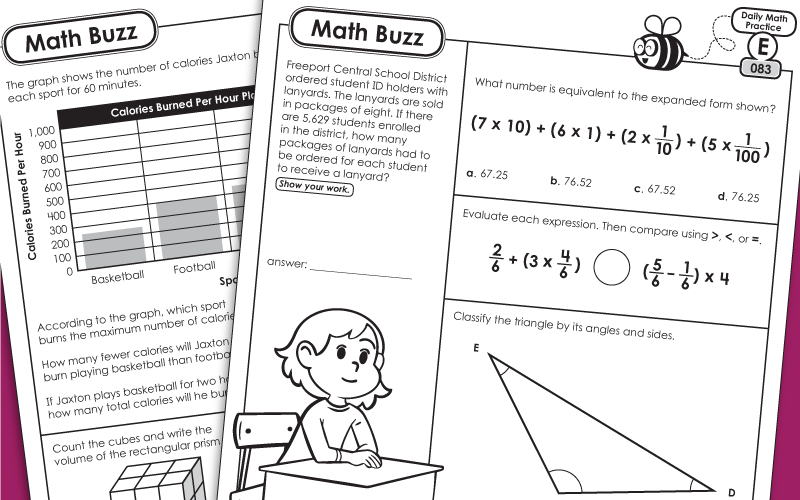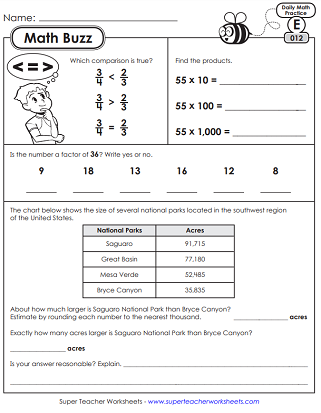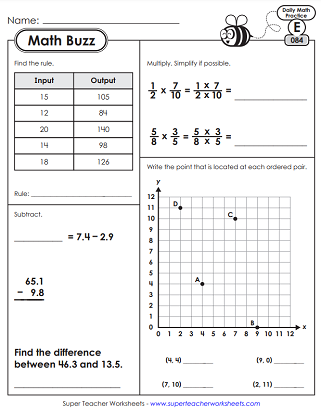# Daily Math Review Worksheets - Level E (5th Grade)

This page contains daily, spiraling math review worksheets for 5th graders. Each file contains 5 worksheets, one for each day of the week. The first few sets review 4th grade content. The later sets challenge students with more advanced 5th grade skills.This is the first week of the 5th grade math buzz series. This file contains 5 worksheets, reviewing basic skills from the fourth grade. Skills covered include: adding and subtracting 5-digit whole numbers, factor pairs, lines of symmetry, rounding, and equivalent fractions.
On these math daily review worksheets, students will review several concepts from last year, including: place value, calculating area, comparing 6-digit numbers, properties of multiplication, and equivalent fractions.
On these printable worksheets, your fifth graders will review parallel/intersecting/perpendicular lines, long division (without remainders), estimating basic operations, factors, area models, and word problems.
Practice drawing parallel line segments, multiplication patterns, comparing fractions, division with remainders, and multiplying 3-digit by 1-digit whole numbers.
Solve math questions related to adding and subtraction fractions (like denominators), multiplying 2-digit by 2-digit numbers, solid shapes, and writing basic algebraic equations.
On these pages, students will solve a 2-step word problem, add mixed numbers (with like denominators), count edges and vertices and solid figures, and compare expressions.
This week, your pupils will work on adding and simplifying fractions, identifying acute/obtuse/right angles, finding the unknown side of a rectangle when given the perimeter, and converting mixed numbers to improper fractions.
Draw an angle with a protractor, add three fractions together and simplify, solve word problems, rewrite phrases as algebraic expressions, convert mixed numbers to fractions, tell whether numbers are prime or composite, and solve long division problems with 4-digit dividends.
On these M.B. worksheets, students will differentiate between prime and composite numbers, calculate measurements of adjacent angles, convert standard measurements, and subtract fractions with unlike denominators.
In the tenth week of Math Buzz, students will use order of operations to evaluate expressions, calculate .1 and 10x a given number, find the volume of a cube, and subtract mixed numbers with unlike denominators.
On these fifth grade math worksheets, students will: answer questions related to a line plot, multiply three-digit numbers by 2-digit numbers, convert linear metric units (mm, cm, m) and multiply fractions by whole numbers.
In the twelfth set, your students will: differentiate between regular and irregular polygons, compare fractions, find area and perimeter of a shape, multiply fractions by whole numbers, and solve word problems.
Find the perimeter of an irregular polygon, write fractions as decimals, classify triangles (scalene, equilateral, isosceles), compare decimals, and review division with remainders.
On this set of Math Buzz worksheets, your students will review: finding the perimeter of an irregular polygon, division with remainders, writing mixed numbers as decimals, multiplying fractions by whole numbers, and using area models to solve problems related to the distributive property of multiplication.
For the fifteenth week, pupils will demonstrate the following mathematical concepts: finding least common multiple (LCM) of two numbers, writing decimal numbers in standard form, multiplying 4-digit by 2-digit numbers, dividing numbers with double-digit divisors, and calculating the volume of a rectangular prism.
On these worksheets, your students can review: decimal place value, triangle classification, multi-digit multiplication and division, reading pie graphs, and solving word problems.
Now that we're into week 17 of 5th grade math buzz, students can review volume of a rectangular prism, multiplication (4-digit by 2-digit) word problems, operations with decimals, multiplying fractions, and plotting points (ordered pairs) on a coordinate grid.
On these five printable daily review worksheets, students will practice calculating greatest common factor (GCF), expressing decimal numbers in expanded form, solving word problems classifying triangles (acute/right/obtuse and isosceles/scalene/equilateral), and operations with decimals.
Review decimal place value (expanded and standard form), multiply by powers of ten, solve operations with decimals, find the area of a rectangle by multiplying a whole number by a fraction.
Plot and label points of a coordinate plane. Interpret a fraction as a division problem. Add, subtract, and multiply decimal numbers. Complete an input/output table and find the rule. Solve single-step and multi-step word problems.
Find the perimeter of a quadrilateral by adding the sides (decimal units). Find the least common multiple (LCM) of a pair of numbers. Answer questions about points on a coordinate grid. Multiply mixed numbers and fractions together. Count cubes to find the volume of an irregular solid.
More 5th grade M.B. activities. Find the perimeter of an irregular quadrilateral by adding the lengths of the sides (decimal units). Multiply mixed numbers and simplify. Evaluate expressions and compare using the symbols <, >, and =. Solve multi-step word problems.
This set has more perimeter, area, and volume problems. There are also a few problems on multiplying and dividing fractions. Students will complete a factor tree. Each worksheet also has a word problem to solve.
Multiply decimals by 10, 100, and 1,000. Add units of time. Evaluate expressions with exponents. Insert parenthesis to make equations true. Divide fractions and simplify.
Answer questions related to: order of operations, converting from kilometers to meters, multiplying decimals, factoring, and long division with decimals.
In week 26 of our 5th grade Math Buzz series, students will: count cubes to determine the volume of a rectangular prism, classify quadrilaterals, solve word problems, and divide by exponents.
This week, students will practice plotting points on a coordinate grid, dividing with decimals, converting gallons to quarts, and finding the volume of an irregular shape.
Multiply decimals by 2-digit numbers, review prime factorization, convert standard units, and calculate the volume of an irregular shape.
Add units of length together (miles and yards). Multiply decimals. Solve equations using the order of operations (including parenthesis and brackets). Use data in a table to write an equation.
This is the final set in the 5th grade Math Buzz series. Write exponents in standard form. Add parenthesis to make equations true (order of operations). Calculate and plot data on a coordinate grid. Solve multi-step word problems.
More Math Buzz Daily Review

STW has daily Math Buzz review for grades 1 through 5. Look here to find Math Buzz worksheets at other the levels.

Daily Word problems

This section of our website has hundreds of Daily Word Problems to challenge your students. Includes 1-step and 2-step problems.

## Sample Worksheet ImagesMy Account
Site Information